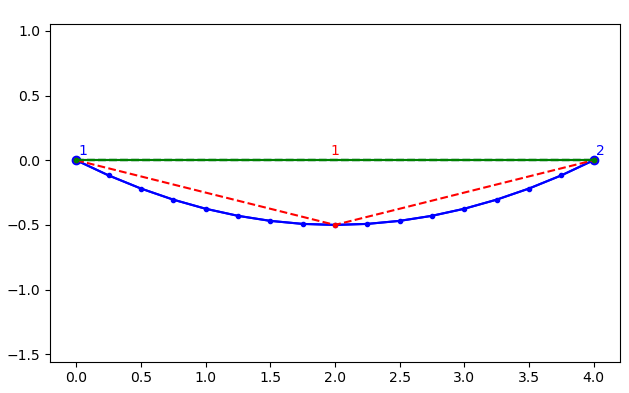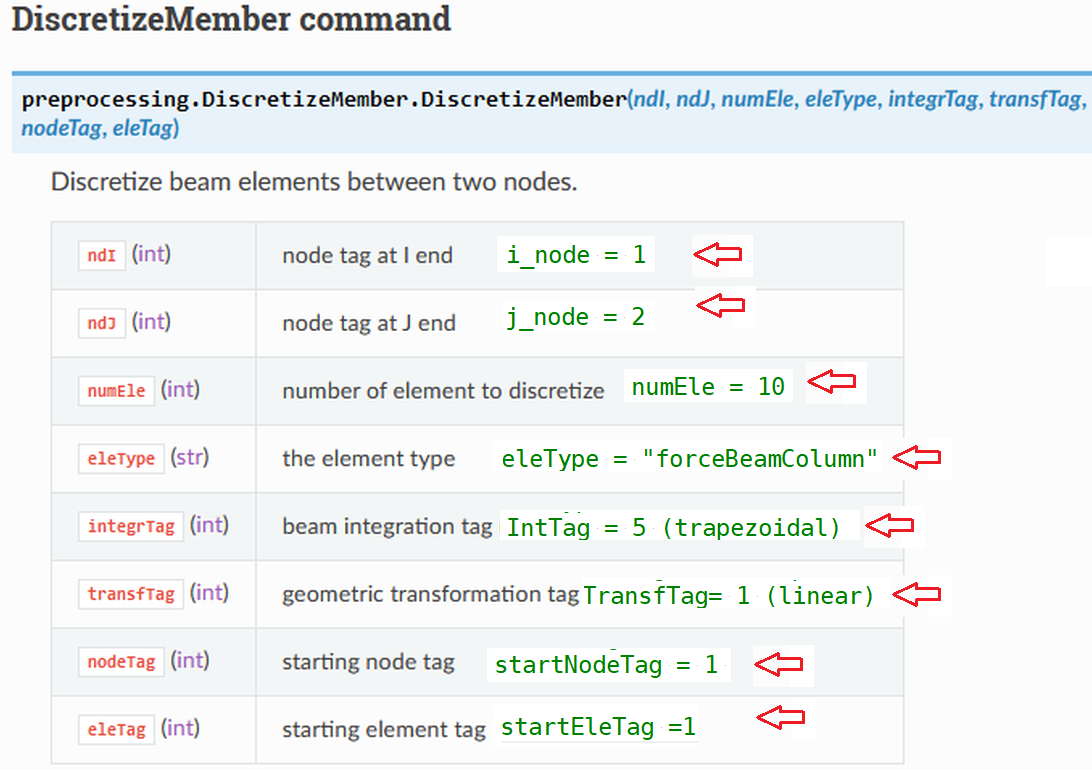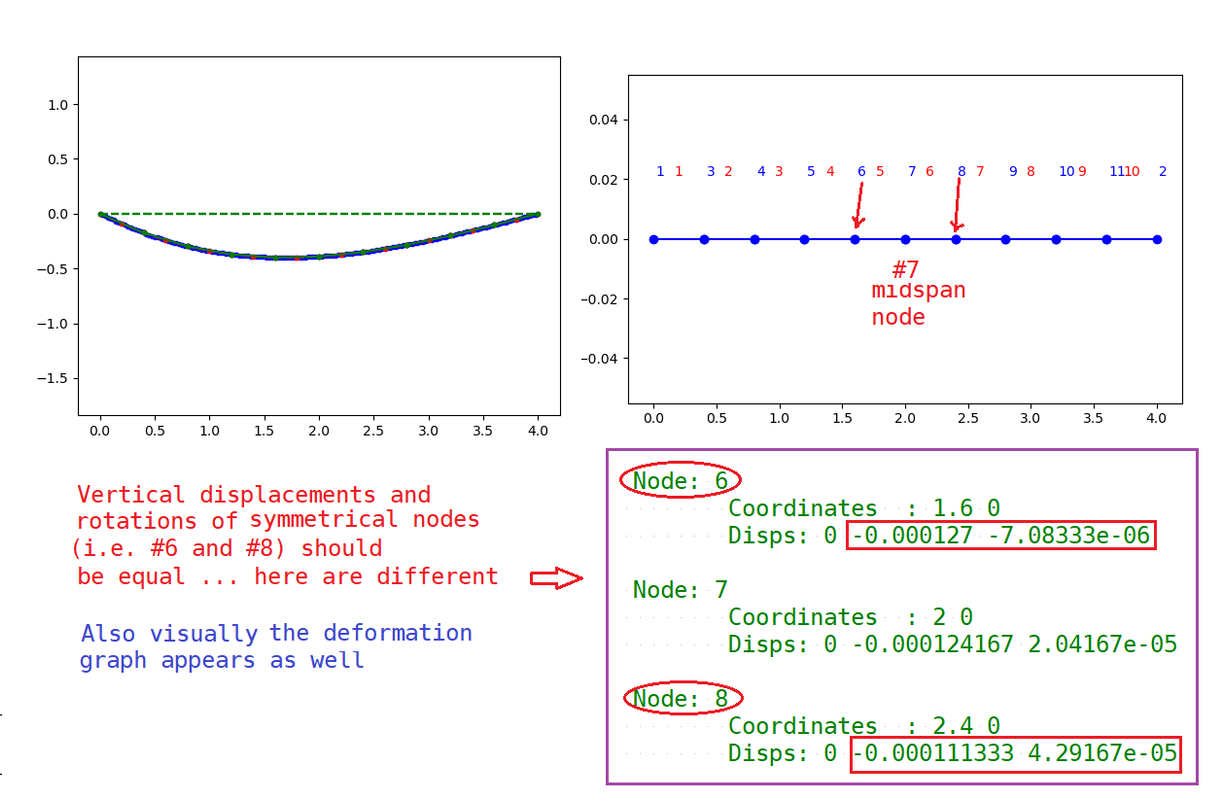## Learning Openseespy through a very simple example [one more good drift, but still failure]

Forum for asking and answering questions related to use of the OpenSeesPy module

Moderators: silvia, selimgunay, Moderators

skypass
Posts: 15
Joined: Thu May 27, 2021 9:37 am

### Learning Openseespy through a very simple example [one more good drift, but still failure]

Hi all,
Just new in this community, please be patient to read what I'm looking for.

Code: Select all

``````https://buildmedia.readthedocs.org/media/pdf/openseespydoc/latest/openseespydoc.pdf
``````
is quite difficult (for newcomers in this module, even being strong in python, like me) to have an immediate vision of such a package.

So, my proposal is: could someone post a .py code to solve this basic problem?

Code: Select all

``````  Given:
* a beam between two simple supports, span L= 4 m
* charged with a distributed load w= 5 kN/m
* having the following geo-mechanics parameters
E= 2e11  pascal # steel modulus of elasticity
I=1.6e-5 m^4    # beam inertial moment
# Neglected section area

Find:
* elastica diagram (plot and deformation values)
spanned 10% (or more) of length L
``````

EDIT: what I'm expecting from Openseespy

Code: Select all

``````# ---- elastica.py -------
L= 4.0 # m
w= 5.0 # kN/m
E= 2e11   # pascal
I= 1.6e-5 # m^4
#
max_displ= 5/384*w*L**4/(E*I) # Elasticity theory
print('\n Max_displacement at midspan=  {:.4e}'.format(max_displ), 'm \n')

Nsteps=17
#
print('{:>7}{:>10}'.format('x', 'eta'))
print('{:>22}'.format('------------------'))
for k in range(Nsteps):
eta= 1.0/(E*I)*(w*(k/L)**4/24 - w*L*(k/L)**3/12 + w*L**3*(k/L)/24 )
print ('{:>9.2f}{:>12.4e}'. format(k/L, eta) )
print('{:>22}'.format('------------------'))
# EOF: elastica.py
'''
Max_displacement at midspan=  5.2083e-06 m

x       eta
------------------
0.00  0.0000e+00
0.25  1.0338e-06
0.50  2.0223e-06
0.75  2.9259e-06
1.00  3.7109e-06
1.25  4.3500e-06
1.50  4.8218e-06
1.75  5.1109e-06
2.00  5.2083e-06
2.25  5.1109e-06
2.50  4.8218e-06
2.75  4.3500e-06
3.00  3.7109e-06
3.25  2.9259e-06
3.50  2.0223e-06
3.75  1.0338e-06
4.00  0.0000e+00
------------------
'''

``````
Last edited by skypass on Sun Jun 06, 2021 8:28 am, edited 6 times in total.

mhscott
Posts: 600
Joined: Tue Jul 06, 2004 3:38 pm
Location: Corvallis, Oregon USA
Contact:

### Re: Learning Openseespy through a very simple example

This is close to what you're looking for: https://portwooddigital.com/2020/11/03/ ... oad-beams/

skypass
Posts: 15
Joined: Thu May 27, 2021 9:37 am

### Re: Learning Openseespy through a very simple example

Oh yes! Thank you, the test below, applied to your suggested code, gives fine results, being known that warning tags need to be deeply investigated.
One more question, please. Which is the command for defining a load uniformly distributed on a beam?
Cheers

Code: Select all

``````Wy = -10.e+3
Wx = 0.
Ew = {3: ['-beamUniform', Wy, Wx]}
``````

Code: Select all

``````C:\Training>python mycode.py
WARNING analysis Static - no Algorithm yet specified,
NewtonRaphson default will be used
WARNING analysis Static - no ConstraintHandler yet specified,
PlainHandler default will be used
WARNING analysis Static - no Numberer specified,
RCM default will be used
WARNING analysis Static - no Integrator specified,
StaticIntegrator default will be used
WARNING analysis Static - no LinearSOE specified,
ProfileSPDLinSOE default will be used

Node 1 reaction=12.5, expected=12.5
Node 2 reaction=-12.5, expected=-12.5
Node 1 rotation=-0.0029556650246305416, expected=-0.002955665024630542
Node 2 rotation=0.005911330049261084, expected=0.005911330049261084
``````

skypass
Posts: 15
Joined: Thu May 27, 2021 9:37 am

### Re: Learning Openseespy through a very simple example

Could someone tell my how to obtain values of displacements, in order to compare with the ones of first post?
Here is the code I've used. Thanks in advance.
Plotted displacements:Code: Select all

``````# --- s.py ---  May.29, 2021 ----
# simply supported beam with distributed load on
#
import openseespy.opensees as ops
import openseespy.postprocessing.ops_vis as opsv

import matplotlib.pyplot as plt

ops.wipe()
ops.model('basic', '-ndm', 2, '-ndf', 3)

L = 4.
A = 2.e-3
I = 1.6e-5

E = 200.e9

Ep = {1: [E, A, I]}

ops.node(1, 0., 0.)
ops.node(2,  L, 0.)

ops.fix(1, 1, 1, 0)  # node 1 soil support
ops.fix(2, 1, 1, 0)  # node 2 soil support

ops.geomTransf('Linear', 1)

ops.element('elasticBeamColumn', 1, 1, 2, A, E, I, 1)
Wy = -5.e+3 # vertical distributed load
Wx = 0.     # horizontal distributed load

Ew = {1: ['-beamUniform', Wy, Wx]}

ops.timeSeries('Constant', 1)
ops.pattern('Plain', 1, 1)

for etag in Ew:
Ew[etag])

ops.constraints('Transformation')
ops.numberer('RCM')
ops.system('BandGeneral')
ops.test('NormDispIncr', 1.0e-6, 6, 2)
ops.algorithm('Linear')
ops.analysis('Static')
ops.analyze(1)

ops.printModel()

szer, wys = 16., 10.

fig = plt.figure(figsize=(szer/2.54, wys/2.54))
ax1 = plt.subplot(111)

opsv.plot_model()

sfac = opsv.plot_defo(120) # factor 120, ok

opsv.plot_defo(sfac, fmt_interp='b.-')
opsv.plot_defo(sfac, 5, interpFlag=0, fmt_nodes='bo-')
opsv.plot_defo(sfac, 3, endDispFlag=0, fmt_interp='r.--')
opsv.plot_defo(sfac, 2, fmt_interp='g.-')

sfacN, sfacV, sfacM = 5.e-5, 5.e-5, 5.e-5

plt.show()

exit()
# EOF: s.py

``````

anuragiitg
Posts: 12
Joined: Mon Aug 26, 2013 7:52 pm
Location: The University of Utah
Contact:

### Re: Learning Openseespy through a very simple example

In order to get displacements at all the intermediate points, you need to divide the beam into multiple elements. Use "DiscretizeMember" from openseespy.preprocessing module.
--

skypass
Posts: 15
Joined: Thu May 27, 2021 9:37 am

### Re: Learning Openseespy through a very simple example

I'll try. Thank you

skypass
Posts: 15
Joined: Thu May 27, 2021 9:37 am

### Re: Learning Openseespy through a very simple example

Around row 70 of s2.py code shown underneath, I summarized the parameters to be filled in (taken from the guide), but
something went wrong, due to lack of experience. At end of the code is visible the error prompt.
Please, could somebody be so kind to write the appropriate parameters inside the command opsdm.DiscretizeMember()?
Thanks.

Code: Select all

``````# --- s2.py ---  Jun.01, 2021 ----
# simply supported beam with distributed load on
# discretizing beam (tentative)
#
import openseespy.opensees as ops
import openseespy.postprocessing.ops_vis as opsv
import openseespy.preprocessing.DiscretizeMember as opsdm

import matplotlib.pyplot as plt

ops.wipe()
ops.model('basic', '-ndm', 2, '-ndf', 3)

L = 4.
A = 2.e-3
I = 1.6e-5

E = 200.e9

Ep = {1: [E, A, I]}

ops.node(1, 0., 0.)
ops.node(2,  L, 0.)

ops.fix(1, 1, 1, 0)  # node 1 soil support
ops.fix(2, 1, 1, 0)  # node 2 soil support

ops.geomTransf('Linear', 1)

ops.element('elasticBeamColumn', 1, 1, 2, A, E, I, 1)
Wy = -5.e+3 # vertical distributed load
Wx = 0.     # horizontal distributed load

Ew = {1: ['-beamUniform', Wy, Wx]}

ops.timeSeries('Constant', 1)
ops.pattern('Plain', 1, 1)

for etag in Ew:
Ew[etag])

ops.constraints('Transformation')
ops.numberer('RCM')
ops.system('BandGeneral')
ops.test('NormDispIncr', 1.0e-6, 6, 2)
ops.algorithm('Linear')
ops.analysis('Static')
ops.analyze(1)

ops.printModel()

szer, wys = 16., 10.

fig = plt.figure(figsize=(szer/2.54, wys/2.54))
ax1 = plt.subplot(111)

opsv.plot_model()
# ----------
opsdm.DiscretizeMember(1, 2, 1, 'elasticBeamColumn', 1, 1, 1, 1)

'''
Discretize beam elements between two nodes.

ndI (int)	node tag at I end
ndJ (int)	node tag at J end
numEle (int)	number of element to discretize
eleType (str)	the element type
integrTag (int)	beam integration tag (beamIntegration commands)
transfTag (int)	geometric transformation tag (geomTransf commands)
nodeTag (int)	starting node tag
eleTag (int)	starting element tag
'''

# ----------

sfac = opsv.plot_defo(120) # factor 120, ok

opsv.plot_defo(sfac, fmt_interp='b.-')
opsv.plot_defo(sfac, 5, interpFlag=0, fmt_nodes='bo-')
opsv.plot_defo(sfac, 3, endDispFlag=0, fmt_interp='r.--')
opsv.plot_defo(sfac, 2, fmt_interp='g.-')

sfacN, sfacV, sfacM = 5.e-5, 5.e-5, 5.e-5

plt.show()

exit()

# EOF: s2.py
'''
Compiling Errors
----------------
SectionForceDeformation *getSectionForceDeformation(int tag) - none found with tag: 1
Traceback (most recent call last):
File "s2.py", line 65, in <module>
opsdm.DiscretizeMember(1, 2, 1, 'elasticBeamColumn', 1, 1, 1, 1)
File "C:\Users\Alfabeta\AppData\Local\Programs\Python\Python38\lib\site-packages\openseespy\preprocessing\Discret
izeMember.py", line 9, in DiscretizeMember
ops.element(eleType,eleTag,ndI,ndJ,transfTag,integrTag)
opensees.OpenSeesError: See stderr output

'''
``````

anuragiitg
Posts: 12
Joined: Mon Aug 26, 2013 7:52 pm
Location: The University of Utah
Contact:

### Re: Learning Openseespy through a very simple example [beam discretization still unsolved]

Have you defined all the parameters needed for the "DiscretizeMember" command? It looks like you haven't defined "Integration Tag" for the element. Also, this command may not work for the "elasticBeamColumn" element. You should use the "forceBeamColumn" or "dispBeamColumn" element with an Elastic section definition.

## Define elastic section using: https://openseespydoc.readthedocs.io/en ... ction.html

section('Elastic', secTag, E_mod, A, Iz, G_mod=None, alphaY=None)

## Then define the elements using the "DiscretizeMember" command. For example, you want to define 10 small equal length elements between node 1 and node 2, with element tag starting with 1.

i_node = 1
j_node = 2
numEle = 10
eleType = "forceBeamColumn"
intTag = 1 (Define it previously. Read the manual and examples to understand what it is.)
transfTag = 1
startNodeTag = 1 (Generally same as i_node)
startEleTag =1

opsdm.DiscretizeMember(i_node, j_node, numEle, eleType, intTag, transfTag, startNodeTag, startEleTag)

## The above command will create all the elements you want between nodes 1 and 2. There is no need to define element 1 separately as you did in your script.
--

anuragiitg
Posts: 12
Joined: Mon Aug 26, 2013 7:52 pm
Location: The University of Utah
Contact:

### Re: Learning Openseespy through a very simple example [beam discretization still unsolved]

This is a great explanation by Dr. Scott.

https://portwooddigital.com/2021/06/02/ ... bers-only/
--

skypass
Posts: 15
Joined: Thu May 27, 2021 9:37 am

### Re: Learning Openseespy through a very simple example [good drift for beam discretization]

Despite the given instructions have been correctly applied (see next screenshot), errors still arise.
New code .py is uploaded below. Thx&Cheers

Code: Select all

``````  File "s3.py", line 65, in <module>
opsdm.DiscretizeMember(1, 2, 10, 'forceBeamColumn', 5, 1, 1, 1)
File "C:\Users\Alfabeta\AppData\Local\Programs\Python\Python38\lib\site-packages\openseespy\preprocessing\Discret
izeMember.py", line 37, in DiscretizeMember
ops.node(nodeTag,Xi+i*dX,Yi+i*dY)
opensees.OpenSeesError: See stderr output
``````File s3.py

Code: Select all

``````# --- s3.py ---  Jun.04, 2021 ----
# simply supported beam with distributed load on
# discretizing beam (2^nd tentative, after forum drift)
#
import openseespy.opensees as ops
import openseespy.postprocessing.ops_vis as opsv
import openseespy.preprocessing.DiscretizeMember as opsdm

import matplotlib.pyplot as plt

ops.wipe()
ops.model('basic', '-ndm', 2, '-ndf', 3)

L = 4.
A = 2.e-3
I = 1.6e-5

E = 200.e9

Ep = {1: [E, A, I]}

ops.node(1, 0., 0.)
ops.node(2,  L, 0.)

ops.fix(1, 1, 1, 0)  # node 1 soil support
ops.fix(2, 1, 1, 0)  # node 2 soil support

ops.geomTransf('Linear', 1)

ops.element('elasticBeamColumn', 1, 1, 2, A, E, I, 1)
Wy = -5.e+3 # vertical distributed load
Wx = 0.     # horizontal distributed load

Ew = {1: ['-beamUniform', Wy, Wx]}

ops.timeSeries('Constant', 1)
ops.pattern('Plain', 1, 1)

for etag in Ew:
Ew[etag])

#ops.constraints('Transformation')
ops.numberer('RCM')
ops.system('BandGeneral')
ops.test('NormDispIncr', 1.0e-6, 6, 2)
#ops.algorithm('Linear')
ops.analysis('Static')
ops.analyze(1)

ops.printModel()

szer, wys = 16., 10.

fig = plt.figure(figsize=(szer/2.54, wys/2.54))
ax1 = plt.subplot(111)

opsv.plot_model()
# ----------
opsdm.DiscretizeMember(1, 2, 10, 'forceBeamColumn', 5, 1, 1, 1)

'''
Parameters of DiscretizeMember() selected as follows:
----------------------------------------------------
i_node = 1
j_node = 2
numEle = 10
eleType = "forceBeamColumn"
IntTag = 5 (trapezoidal)
TransfTag= 1 (linear)
startNodeTag = 1
startEleTag =1
'''

sfac = opsv.plot_defo(120) # factor 120, ok

opsv.plot_defo(sfac, fmt_interp='b.-')
opsv.plot_defo(sfac, 5, interpFlag=0, fmt_nodes='bo-')
opsv.plot_defo(sfac, 3, endDispFlag=0, fmt_interp='r.--')
opsv.plot_defo(sfac, 2, fmt_interp='g.-')

sfacN, sfacV, sfacM = 5.e-5, 5.e-5, 5.e-5

plt.show()

exit()

# EOF: s3.py
'''
C:\Training>python s3.py
Current Domain Information
Current Time: 1
tCommitted Time: 1
NODE DATA: NumNodes: 2

numComponents: 2
Node: 1
Coordinates  : 0 0
Disps: 0 0 -0.00416667
ID : -1 -1 1

Node: 2
Coordinates  : 4 0
Disps: 0 0 0.00416667
ID : -1 -1 0

ELEMENT DATA: NumEle: 1

numComponents: 1
ElasticBeam2d: 1
Connected Nodes: 1 2
CoordTransf: 1
mass density:  0, cMass: 0
release code:  0
End 1 Forces (P V M): 0 10000 0
End 2 Forces (P V M): 0 10000 0

SP_Constraints: numConstraints: 4

numComponents: 4SP_Constraint: 0         Node: 1 DOF: 1 ref value: 0 current value: 0
SP_Constraint: 1         Node: 1 DOF: 2 ref value: 0 current value: 0
SP_Constraint: 2         Node: 2 DOF: 1 ref value: 0 current value: 0
SP_Constraint: 3         Node: 2 DOF: 2 ref value: 0 current value: 0

Pressure_Constraints: numConstraints: 0

numComponents: 0
MP_Constraints: numConstraints: 0

numComponents: 0

Scale Factor: 1
Constant Series: factor: 1

numComponents: 0

Transverse: -5000
Axial:      0
Element acted on: 1

Single Point Constraints:

numComponents: 0
PARAMETERS: numParameters: 0

WARNING: failed to add node to domain
Traceback (most recent call last):
File "s3.py", line 65, in <module>
opsdm.DiscretizeMember(1, 2, 10, 'forceBeamColumn', 5, 1, 1, 1)
File "C:\Users\Alfabeta\AppData\Local\Programs\Python\Python38\lib\site-packages\openseespy\preprocessing\Discret
izeMember.py", line 37, in DiscretizeMember
ops.node(nodeTag,Xi+i*dX,Yi+i*dY)
opensees.OpenSeesError: See stderr output
'''
``````

anuragiitg
Posts: 12
Joined: Mon Aug 26, 2013 7:52 pm
Location: The University of Utah
Contact:

### Re: Learning Openseespy through a very simple example [good drift for beam discretization, code still fails]

Here is a minimum working script for you,

Code: Select all

``````import openseespy.opensees as ops
import openseespy.preprocessing.DiscretizeMember as opsdm

import matplotlib.pyplot as plt

ops.wipe()
ops.model('basic', '-ndm', 2, '-ndf', 3)

L = 4.
A = 2.e-3
I = 1.6e-5

E = 200.e9

Ep = {1: [E, A, I]}

ops.node(1, 0., 0.)
ops.node(2,  L, 0.)

ops.fix(1, 1, 1, 0)  # node 1 soil support
ops.fix(2, 1, 1, 0)  # node 2 soil support

ops.geomTransf('Linear', 1)

SecTag = 1
IntTag = 1

ops.section('Elastic', SecTag, E, A, I)
ops.beamIntegration('Lobatto', IntTag, SecTag, 4)

#ops.element('elasticBeamColumn', 1, 1, 2, A, E, I, 1)

opsdm.DiscretizeMember(1, 2, 10, 'forceBeamColumn', IntTag, 1, 3, 1)

'''
Parameters of DiscretizeMember() selected as follows:
----------------------------------------------------
i_node = 1
j_node = 2
numEle = 10
eleType = "forceBeamColumn"
IntTag = 5 (trapezoidal)
TransfTag= 1 (linear)
startNodeTag = 1
startEleTag =1
'''

Wy = -5.e+3 # vertical distributed load
Wx = 0.     # horizontal distributed load

Ew = {1: ['-beamUniform', Wy, Wx]}

ops.timeSeries('Constant', 1)
ops.pattern('Plain', 1, 1)

for etag in Ew:
Ew[etag])

``````
--

skypass
Posts: 15
Joined: Thu May 27, 2021 9:37 am

### Re: Learning Openseespy through a very simple example [good drift for beam discretization, code still fails]

Thanks so much, anuragiitg. Again something doesn't go properly, according to the following screenshot and the code s5.py below, derived from your drift. Prompt on opening post title: "one more good drift, but still failure"file s5.py

Code: Select all

``````# --- s5.py --- Jun.06. 2021
import openseespy.opensees as ops
import openseespy.preprocessing.DiscretizeMember as opsdm
import openseespy.postprocessing.ops_vis as opsv

import matplotlib.pyplot as plt

ops.wipe()
ops.model('basic', '-ndm', 2, '-ndf', 3)

L = 4.
A = 2.e-3
I = 1.6e-5

E = 200.e9

Ep = {1: [E, A, I]}

ops.node(1, 0., 0.)
ops.node(2,  L, 0.)

ops.fix(1, 1, 1, 0)  # node 1 soil support
ops.fix(2, 1, 1, 0)  # node 2 soil support

ops.geomTransf('Linear', 1)

SecTag = 1
IntTag = 1

ops.section('Elastic', SecTag, E, A, I)
ops.beamIntegration('Lobatto', IntTag, SecTag, 4)

#ops.element('elasticBeamColumn', 1, 1, 2, A, E, I, 1)

opsdm.DiscretizeMember(1, 2, 10, 'forceBeamColumn', IntTag, 1, 3, 1)

Wy = -5.e+3 # vertical distributed load
Wx = 0.     # horizontal distributed load

Ew = {1: ['-beamUniform', Wy, Wx]}

ops.timeSeries('Constant', 1)
ops.pattern('Plain', 1, 1)

for etag in Ew:
Ew[etag])

# ---- Piece of code added to the above suggestion -------
ops.constraints('Transformation')
ops.numberer('RCM')
ops.system('BandGeneral')
ops.test('NormDispIncr', 1.0e-6, 6, 2)
ops.algorithm('Linear')
ops.analysis('Static')
ops.analyze(1)

ops.printModel()

# plot model with tag lebels

szer, wys = 16., 10.

fig = plt.figure(figsize=(szer/2.54, wys/2.54))
ax1 = plt.subplot(111)

opsv.plot_model()
sfacN, sfacV, sfacM = 5.e-1, 5.e-1, 5.e-1 # scale factors

# plot deformed model
plt.figure()
# plot_defo with optional arguments
sfac = opsv.plot_defo() #
print(f'sfac: {sfac}')  # return sfac if automatically calculated

opsv.plot_defo(sfac, fmt_interp='b.-')
opsv.plot_defo(sfac, 5, interpFlag=0, fmt_nodes='bo-')
opsv.plot_defo(sfac, 3, endDispFlag=0, fmt_interp='r.--')
opsv.plot_defo(sfac, 2, fmt_interp='g.-')

# ----------
plt.show()
# ----------

exit()
# EOF: s5.py
'''
numComponents: 11
Node: 1
Coordinates  : 0 0
Disps: 0 0 -0.000150417

Node: 3
Coordinates  : 0.4 0
Disps: 0 -5.55e-05 -0.000119583

Node: 4
Coordinates  : 0.8 0
Disps: 0 -9.46667e-05 -7.70833e-05

Node: 5
Coordinates  : 1.2 0
Disps: 0 -0.000117833 -3.95833e-05

Node: 6
Coordinates  : 1.6 0
Disps: 0 -0.000127 -7.08333e-06

Node: 7
Coordinates  : 2 0
Disps: 0 -0.000124167 2.04167e-05

Node: 8
Coordinates  : 2.4 0
Disps: 0 -0.000111333 4.29167e-05

Node: 9
Coordinates  : 2.8 0
Disps: 0 -9.05e-05 6.04167e-05

Node: 10
Coordinates  : 3.2 0
Disps: 0 -6.36667e-05 7.29167e-05
ID : 4 5 6

Node: 11
Coordinates  : 3.6 0
Disps: 0 -3.28333e-05 8.04167e-05

Node: 2
Coordinates  : 4 0
Disps: 0 0 8.29167e-05